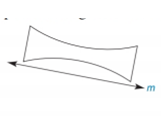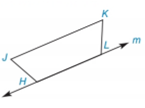Chapter 2.6, Problem 14E### Elementary Geometry for College St...

6th Edition
Daniel C. Alexander + 1 other
ISBN: 9781285195698

#### Solutions

Chapter
Section### Elementary Geometry for College St...

6th Edition
Daniel C. Alexander + 1 other
ISBN: 9781285195698
Textbook Problem
1 views

# Complete each figure so that it reflects across line m . a)b)To determine

a)

To draw:

The complete figure symmetrical to line m.

Explanation

Definition Used:

The figure to be reflected across the given line this implies that for every point A on the left side of the line, there should be second point A such that line should act as the perpendicular bisector of AA¯

To determine

b)

To draw:

The complete figure symmetrical to line m.

### Still sussing out bartleby?

Check out a sample textbook solution.

See a sample solution

#### The Solution to Your Study Problems

Bartleby provides explanations to thousands of textbook problems written by our experts, many with advanced degrees!

Get Started

#### In Exercises 2528. solve the equation by factoring. 27. x3 2x2 + 3x = 0

Applied Calculus for the Managerial, Life, and Social Sciences: A Brief Approach

#### Simplify the expressions in Exercises 97106. 32/331/6

Finite Mathematics and Applied Calculus (MindTap Course List)

#### In Problems 51 and 52, use a change-of-base formula to evaluate each logarithm.

Mathematical Applications for the Management, Life, and Social Sciences

#### Convert from radians to degrees. 12. 5

Single Variable Calculus: Early Transcendentals, Volume I

#### Find the limit: limx1(2x2x+4).

Calculus: An Applied Approach (MindTap Course List)

#### True or False: 23xdx is an improper integral.

Study Guide for Stewart's Single Variable Calculus: Early Transcendentals, 8th

#### Distinguish between a hypothesis and a prediction.

Research Methods for the Behavioral Sciences (MindTap Course List)